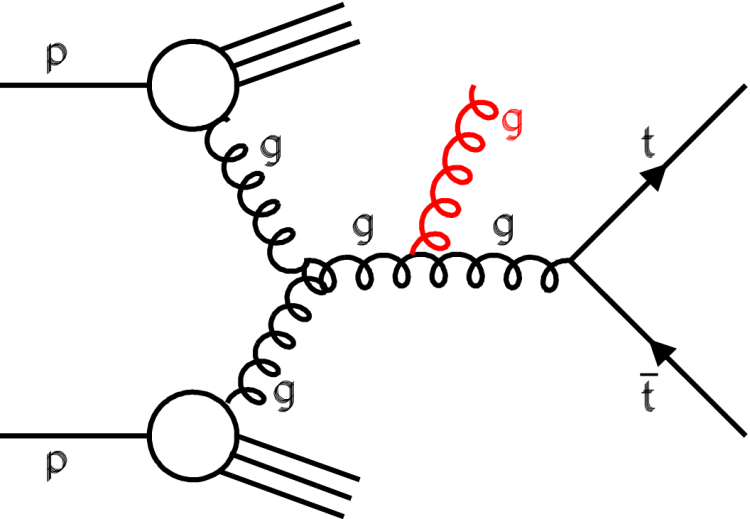•The CMS experiment

•a graphical representation of an LHC collision, which is read from left to right. The gluons (g) from the protons (p) collide, merge and create a top quark-antiquark pair. Extra gluons (red) can also be created.

For the first time, CMS physicists have measured the production rate of top quark pairs as a function of three experimental observables simultaneously. The new result helps to answer several fundamental questions, among which, the properties of gluons inside protons, the strength of the strong nuclear interaction, and how massive the top quark precisely is.

The top quark is a mysterious elementary particle.  It is by far more massive than all other known quarks (up, down, strange, charm and bottom) and it is not understood why this is so. At the LHC, studying the top quark has become routine operation. Just during 2016, more than 30 million top quarks were produced by the pp collisions inside the CMS detector.  This number is so large because of the strong force that acts when two protons collide. Gluons are the particles that mediate the strong force, and they are particularly abundant in the high energy LHC protons. The Standard Model of particle physics describes the dominant reaction for top quark production at the LHC: the interaction of a gluon from one proton with a gluon from the other proton to produce a pair of a top and anti-top quark out of the vacuum.Figure 1: a graphical representation of an LHC collision, which is read from left to right. The gluons (g) from the protons (p) collide, merge and create a top quark-antiquark pair. Extra gluons (red) can also be created.

Figure 1 shows a graphical representation of what happens during the production of a top quark and its antiquark, this is the most common way to produce top quarks at the LHC. It turns out that, by studying selected directional and energy information in these events, one can answer several fundamental physics questions.

#### 1. Understanding the proton better

Understanding the properties of gluons inside protons is essential for predictions of many high energy transfer reactions at the LHC. This knowledge is also used to predict the frequency and properties of the signatures of possible new massive particles.  Experimentally this can be answered by studying the distributions of the invariant mass of the top quark-antiquark system and its direction with respect to the LHC beam (the quantity used is called rapidity). Studying the rapidity and invariant mass of top quark pairs can give physicists information about the dependence of the gluon density as what fraction of the gluon comes from the proton momentum. The gluon density can be measured in other ways as well, but top quarks are used now to also study the gluons in the proton at relatively large fractions of the proton momentum up to 30% of the LHC proton energy!

#### 2. Understanding the top quark mass and its link to the stability of the universe

Another question that can be answered by detailed studies of the top quark is what its exact mass is. Knowing the top quark mass value as accurately as possible is of extreme importance since, from our current knowledge, even the long-term stability of the universe depends crucially on the exact value.  The question can be tackled experimentally by studying the distribution of the invariant mass of the top quark-antiquark system. CMS physicists have developed a new technique that enables more precise measurement of what happens when top quark-antiquark pairs are produced near the lowest allowed energy, at twice the top quark mass. The extraction of the top pole mass, defined as the mass of a particle at rest, from the mass spectrum of top quark pairs is the first of its kind.

#### 3. Understanding how strong the strong force is

The strong force is by far stronger than any other force we know. The strength is expressed by a number called the strong coupling strength, which is a fundamental constant of nature. The strong coupling strength also defines the chance that an additional hard gluon is created in LHC collisions, in Figure 1, such a gluon is shown as a red helix. Radiation of gluons or quarks leads to a collimated spray of particles in the detector, which is called a jet.  CMS physicists have for the first time used the number of additional jets in top-quark pair events to extract the strong coupling constant.

All the above fundamental questions are tackled in one go in a recent measurement of the production probability of top quark pairs at the LHC, which in particle physics is called a cross-section. The most reliable way to select purely top-quark pairs was used, which involves requiring an electron, a muon, and extra jets. This selection resulted in a nearly background-free event sample of about 250000 top quark pair events. CMS physicists used that sample and have measured the cross section as a function of all three observables: the invariant mass and rapidity of the top quark-antiquark pair system and the number of additional jets. The measurement is repeated in 24 different ranges of the three variables.

The new results significantly improve the knowledge of the gluon density at large proton momentum fractions and at the same time allow accurate determination of the top quark pole mass and the strong coupling strength. In particular, the top pole mass determination is noteworthy, with an uncertainty of ~0.8 GeV (that is about 0.45% precision), and that is competitive with the uncertainty of the average world value that combines many separate results from different experiments.

Measuring the properties of a particle such as the top quark in this much detail takes time and relies on very detailed understanding of the data collected by the CMS experiment. While this result uses the data collected in 2016, CMS physicists are already working on improving these results even further with the dataset from Run 2 of the LHC.